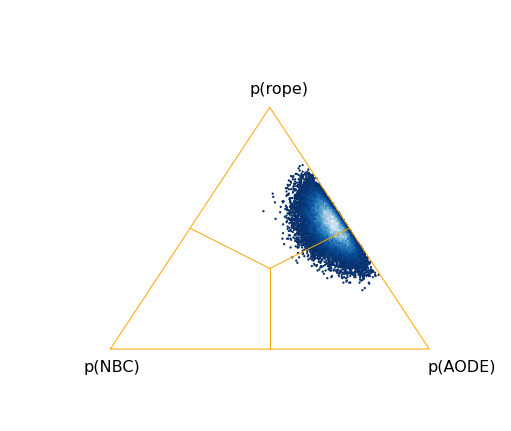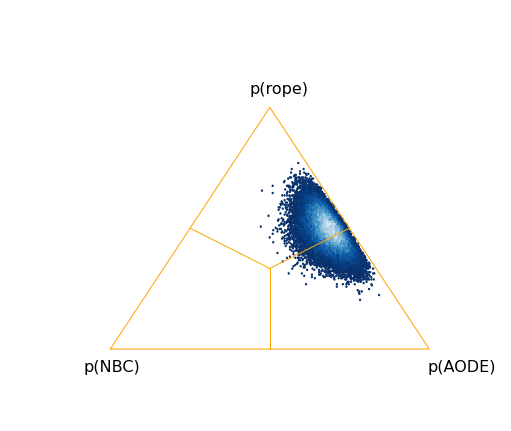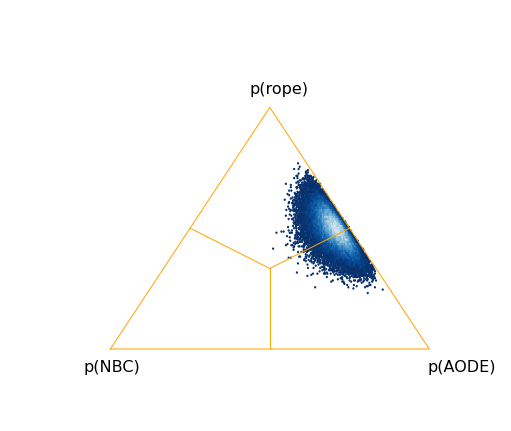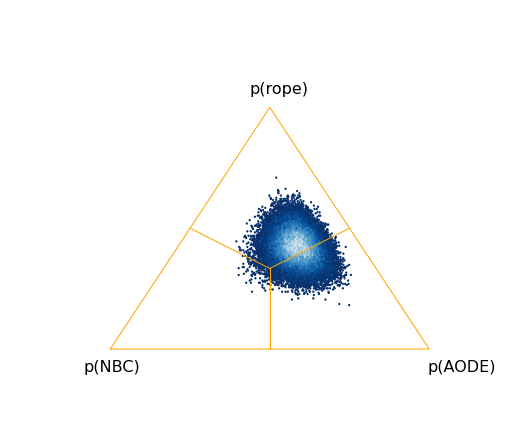## Monday, November 14, 2016

### New Blog address

I moved my blog here alessiobenavoli.com/blog/

## Tuesday, November 1, 2016

### Clinton's win is still certain

Using the latest poll data, it seems clear that Clinton's win is almost certain.
On average she will get between 320 (worst case) to 333 (best case) electoral votes. So if something is going to change, then it is not in the poll data yet.

## Friday, October 28, 2016

### 28 October, USA general election situation

These are the updated probability obtained running the Python code that computes the Bayesian posterior distribution over the electoral votes using near-ignorance priors. The worst and best case distribution for Clinton are in red and respectively, blue..

• Winning probability above 0.99 (for both worst and best scenario).
• Electoral votes between 322 and 335 (mean of the worst and best distribution)

The state-by-state situation is here

## Sunday, October 23, 2016

### Quantum Fréchet bounds

If you are interested in the Quantum Mechanics version of Fréchet bounds, then I have just edited the Fréchet inequalities page in wikipedia to show that similar bounds can also be obtained in quantum mechanics for separable density matrices. These bounds were derived in our paper:

A. Benavoli, A. Facchini and M. Zaffalon. Quantum mechanics: The Bayesian theory generalized to the space of Hermitian matrices. Phys. Rev. A, 94:042106 , 1-27, 2016.

It is worth to point out that entangled states violate these Fréchet bounds. Entangled states exhibit a form of stochastic dependence stronger than the strongest classical dependence and in fact they violate Fréchet like bounds. Another example of violation of probabilistic bounds is provided by the famous Bell's inequality.

## Sunday, October 16, 2016

### Bayesian Sign Test

This post explains how to perform a Bayesian sign test for comparing the performance of two classifiers on different datasets. This module is part of a tool that I am developing together with Giorgio Corani and Janez Demsar. The code of this Ipython Notebook and the code of the test can be found here

# Bayesian Sign Test

Module signtest in bayesiantests computes the probabilities that, based on the measured performance, one model is better than another or vice versa or they are within the region of practical equivalence.

This notebook demonstrates the use of the module.

We will load the classification accuracies of the naive Bayesian classifier and AODE on 54 UCI datasets from the file Data/accuracy_nbc_aode.csv. For simplicity, we will skip the header row and the column with data set names.

In :
import numpy as np
scores = np.loadtxt('Data/accuracy_nbc_aode.csv', delimiter=',', skiprows=1, usecols=(1, 2))
names = ("NBC", "AODE")


Functions in the module accept the following arguments.

• x: a 2-d array with scores of two models (each row corresponding to a data set) or a vector of differences.
• rope: the region of practical equivalence. We consider two classifiers equivalent if the difference in their performance is smaller than rope.
• prior_strength: the prior strength for the Dirichlet distribution. Default is 1.
• prior_place: the region into which the prior is placed. Default is bayesiantests.ROPE, the other options are bayesiantests.LEFT and bayesiantests.RIGHT.
• nsamples: the number of Monte Carlo samples used to approximate the posterior.
• names: the names of the two classifiers; if x is a vector of differences, positive values mean that the second (right) model had a higher score.

### Summarizing probabilities

Function signtest(x, rope, prior_strength=1, prior_place=ROPE, nsamples=50000, verbose=False, names=('C1', 'C2')) computes the Bayesian sign test and returns the probabilities that the difference (the score of the first classifier minus the score of the first) is negative, within rope or positive.

In :
import bayesiantests as bt
left, within, right = bt.signtest(scores, rope=0.01)
print(left, within, right)

0.0 0.71288 0.28712


The first value (left) is the probability that the first classifier (the left column of x) has a higher score than the second (or that the differences are negative, if x is given as a vector).

In the above case, the right (AODE) performs worse than naive Bayes with a probability of 0.29, and they are practically equivalent with a probability of 0.71.

If we add arguments verbose and names, the function also prints out the probabilities.

In :
left, within, right = bt.signtest(scores, rope=0.01, verbose=True, names=names)

P(NBC > AODE) = 0.0, P(rope) = 0.70982, P(AODE > NBC) = 0.29018


The posterior distribution can be plotted out:

1. using the function signtest_MC(x, rope, prior_strength=1, prior_place=ROPE, nsamples=50000) we generate the samples of the posterior
2. using the function plot_posterior(samples,names=('C1', 'C2')) we then plot the posterior in the probability simplex
In :
%matplotlib inline
import matplotlib.pyplot as plt

samples = bt.signtest_MC(scores, rope=0.01)

fig = bt.plot_posterior(samples,names)

plt.show()### Checking sensitivity to the prior

To check the effect of the prior, let us a put a greater prior on the left.

In :
samples = bt.signtest_MC(scores, rope=0.01,  prior_strength=1, prior_place=bt.LEFT)
fig = bt.plot_posterior(samples,names)
plt.show()... and on the right

In :
samples = bt.signtest_MC(scores, rope=0.01,  prior_strength=1, prior_place=bt.RIGHT)
fig = bt.plot_posterior(samples,names)
plt.show()The prior with a strength of 1 has negligible effect. Only a much stronger prior on the left would shift the probabilities toward NBC:

In :
samples = bt.signtest_MC(scores, rope=0.01,  prior_strength=10, prior_place=bt.LEFT)
fig = bt.plot_posterior(samples,names)
plt.show()## Auxiliary functions

The function signtest_MC(x, rope, prior_strength=1, prior_place=ROPE, nsamples=50000) computes the posterior for the given input parameters. The result is returned as a 2d-array with nsamples rows and three columns representing the probabilities $p(-\infty, rope), p[-rope, rope], p(rope, \infty)$. Call signtest_MC directly to obtain a sample of the posterior.

The posterior is plotted by plot_simplex(points, names=('C1', 'C2')), where points is a sample returned by signtest_MC.

@ARTICLE{bayesiantests2016, author = {{Benavoli}, A. and {Corani}, G. and {Demsar}, J. and {Zaffalon}, M.}, title = "{Time for a change: a tutorial for comparing multiple classifiers through Bayesian analysis}", journal = {ArXiv e-prints}, archivePrefix = "arXiv", eprint = {1606.04316}, url={https://arxiv.org/abs/1606.04316}, year = 2016, month = jun }